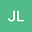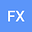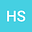Influence of Initial Crack-depth Ratio on Dynamic Fracture Characteristics of FRP Reinforced Concrete
•••• Jueding Liu,
• fnq xiangqian,
• hu shaowei
Jueding Liu
Nanjing Hydraulic Research Institute
Author Profilefnq xiangqian
Author Profilehu shaowei
Nanjing Hydraulic Research Institute
Author Profile## Abstract

Abstract: In order to study the dynamic fracture characteristics of FRP reinforced concrete, the three-point bending experiment of FRP reinforced concrete beams with different initial crack-depth ratios was carried out by using MTS-810 hydraulic servo experiment machine. The initial crack-depth ratios are 0.2, 0.3, 0.4, 0.5 and 0.6 respectively. The effects of different initial crack-depth ratios on the load-crack opening displacement curve, failure process, the increment of critical crack-depth ratio and flexibility coefficient of FRP reinforced concrete three-point bending beam specimens were analyzed by displacement controlled monotonic loading method. Combined with Pearson correlation experiment formula, the correlation between initial crack-depth ratios and different fracture parameters was quantitatively analyzed. The conclusions can be drawn that, there are three obvious critical points in the fracture process of FRP reinforced concrete three-point bending beam: the starting point of concrete crack, the first peak point and the ultimate bearing capacity point. With the increase of the initial crack-depth ratio, the crack initiation load decreases, but the first peak load and ultimate load increase first and then decrease. It is found that the stress intensity factors of the three critical points increase first and then decrease with the increase of the initial crack-depth ratio, and when the initial crack-depth ratio is 0.4, the three kinds of stress intensity factors all reach the maximum value. The increment of critical crack-depth ratio and flexibility coefficient of FRP reinforced concrete beams are related to the initial crack-depth ratio, which can be used to reflect the influence of initial crack-depth ratio on the deformation capacity of concrete specimens. Keywords: FRP reinforced concrete; dynamic fracture; the initial crack-depth ratios; stress intensity factors; increment of critical crack-depth ratio; flexibility coefficient